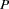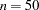The GENESELECT Procedure

PROC GENESELECT <options> ;

The PROC GENESELECT statement starts the GENESELECT procedure. Either the DATA= option or the INMODEL= option must appear, but not both. The DATA= option must appear in order to fit a model. The INMODEL= option specifies a previously saved model. Table 7.2 summarizes the options available in the PROC GENESELECT statement.

Table 7.2 PROC GENESELECT Statement Options

Option

Description

Default

DATA=

data set

INMODEL=

data set containing model information

LEAFFRACTION=

LEAFSIZE as fraction of data

0.001

LEAFSIZE=

minimum number of observations in a branch

MAXDEPTH=

maximum depth of a tree

6

MAXSURROGATES=

maximum number of surrogates rules in a node

10

MINCATSIZE=

observations needed for each category

5

MODELTYPE=

type of model to fit

TREEBOOST

SEED=

seed for pseudo-random number generator

8976153

SPLITSIZE=

minimum number of observations to split a node

10

DATA=SAS-data-set

specifies data. Either the DATA= or INMODEL= option must be specified, but not both.

INMODEL=SAS-data-set

names a data set created from the SAVE MODEL= option. When using the INMODEL= option, the VAR, TRAIT, and FREQ statements are prohibited, as are the DATA= option and other model parameters.

ITERATIONS=n

specifies the number of terms in a boosted series of trees. For quantitative and binary traits, the number of iterations equals the number of trees. For a qualitative, nonbinary trait, a separate tree is created for each trait category in each iteration, resulting intrees, whereis the number of trait values andis an integer from 1 to 1000. The default value ofis 50.

LEAFFRACTION=p

specifies the smallest number of observations a new branch can have, expressed as the proportion of the numberof available observations in the DATA= data set specified in the PROC statement.can be less than the total number of observations in the data set because observations with a missing trait or nonpositive value of the variable specified in the FREQ statement are excluded from. The LEAFSIZE= option specifies the same quantity as an absolute number. The procedure uses the larger of the two.can be any number from zero through one. The default value equals 0.001.

LEAFSIZE=n

specifies the smallest number of observations a new branch can have. The LEAFFRACTION= option specifies the same quantity as a proportion of the original data. The procedure uses the larger of the two. The default value equals the numberof available observations in the DATA= data set specified in the PROC statement, divided by 1,000, or 5, if 5 is larger, or 5,000, if 5,000 is smaller.can be less than the total number of observations in the data set because observations with a missing trait or nonpositive value of the variable specified in the FREQ statement are excluded from. The LEAFSIZE= option does not use the values of the variable in the FREQ statement to adjust the count of observations in the leaf.

MAXDEPTH=n | MAX

specifies the maximum depth of a node that the PROC statement creates automatically. The depth of a node equals the number of splitting rules needed to define the node. The root node has a depth of zero. The children of the root have a depth of one, and so on.

The MAXDEPTH=MAX option specifies, the largest possible value of. The smallest acceptable value ofis 0. Specify MAXDEPTH=0 to avoid creating a model. The default value ofdepends on the MODELTYPE= option. The default value ofis six for MODELTYPE=TREE, and two for MODELTYPE= TREEBOOST.

MAXSURROGATES | MAXSURRS=n

specifies the number of surrogate rules sought for each internal node. A surrogate rule is one that emulates the splitting rule. The measure of agreement between a surrogate and the splitting rule is used to compute the interchangeability of two variables. The GENESELECT procedure does not use surrogate rules to predict observations with missing values. The default value ofis 10.

MINCATSIZE=n

specifies the minimum number of observations that a given qualitative variable value must have in order to use the value in a split search. The GENESELECT procedure handles qualitative values that appear in fewer thanobservations in the same way it handles missing values. The default value ofis 5.

MODELTYPE=type

specifies the type of model to create. Table 7.3 summarizes the types available.

Table 7.3 Types of Models

Type

Description

TREEBOOST

Boosted series of trees

TREE

Decision tree

SEED=n

specifies the seed for generating random numbers. The value specified formust be a nonnegative integer. Setto 0 to use the internal default.

SPLITSIZE=n

specifies the requisite number of observations a node must have in order for the procedure to consider splitting it. By default,is twice the value of the LEAFSIZE= option. For the LEAFFRACTION=, LEAFSIZE=, MINCATSIZE=, and SPLITSIZE= options in the PROC statement, and for the NODESIZE= option in the PERFORMANCE statement, the procedure counts the number of observations in a node without adjusting the number with the values of the variable specified in the FREQ statement.

Note: This procedure is experimental.Previous Page | Next Page | Top of Page